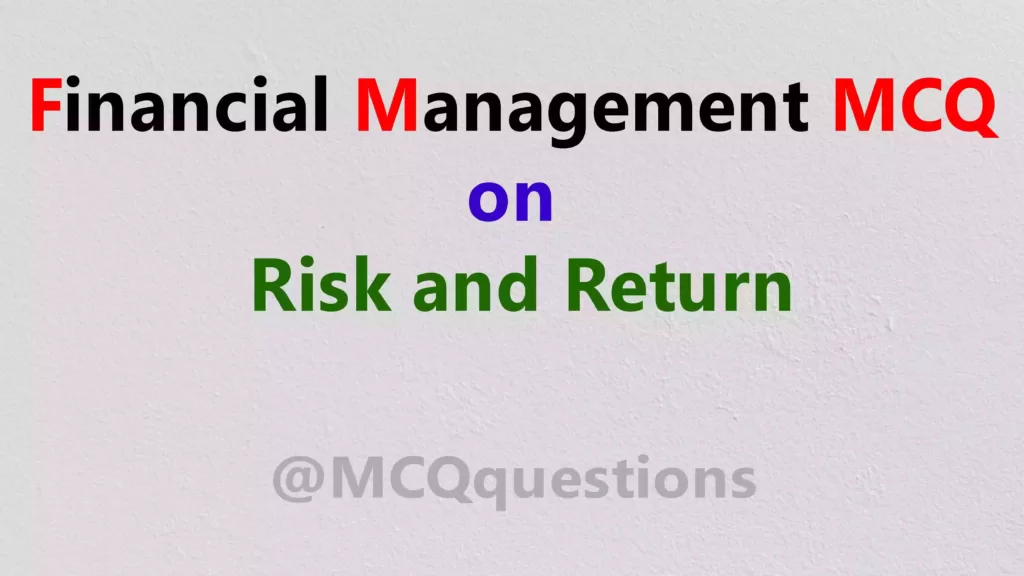# Financial Management MCQ on Risk and Return

## Financial Management MCQ on Risk and Return

1. The degree correlation between risk and return over a longer period of time is generally believed to be as –

(a) Highly negative

(b) Negative

(c) Highly positive

(d) No correlation

Ans-(c) Highly positive

2. The appreciation in the value of security or asset is called as:

(a) Current return

(b) Total return

(c) Capital return

(d) None of these

Ans-(c) Capital return

3. The price of a security at the beginning of year is 100, the price at the end of the year is 125 and dividend paid at the end of the year is Rs.5. The current return of the security is:

(a) 2.5%

(b) 5%

(c) 10%

(d) 15%

Ans-(b) 5%

4. Current return is the ratio of annual income to:

(a) Ending price of security

(b) Beginning price of security

(c) Difference between beginning price and ending price of security

(d) Total of beginning price and ending price of security

Ans-(b) Beginning price of security

5. The price of a security at the beginning of year is 200, the price at the end of the year is 225 and dividend paid at the end of the year is 15. The total rate of return is:

(a)10%

(b) 20%

(c) 25%

(d) 15%

Ans-(b) 20%

6. The application of the probability distribution is the characteristic of possible outcome that must be:

(a) Mutually inclusive

(b) Collective exhausting

(c) Either collective exhausting or mutually inclusive

(d) None of these

Ans-(d) None of these

7. The minimum expected rate of return that is needed to persuade an investor to purchase the security at given risk is which of the following ?

(b) Risk free rate of return

(c) Required rate of return

Ans-(c) Required rate of return

8. The risk that arises due to use of debt by the firm causing variability of return for creditors and shareholders is:

(a) Call Risk

(b) Liquidity Risk

(c) Financial Risk

(d) Default Risk

Ans-(c) Financial Risk

9. The variability in return on security due to changes in the level of interest rate in

market is called as:

(a) Call Risk

(b) Interest Risk

(c) Liquidity Risk

(d) Financial Risk

Ans-(b) Interest Risk

10. Cumulative wealth index measures the level of wealth of investor by taking the cumulative effect of:

(a) Dividend

(c) Total risk

(d) Total return

Ans-(d) Total return

11. Purchasing power of risk hit the investor when there are chances that the:

(a) Real return on a security is less than the nominal return

(b) Real return on a security is equal to the nominal return

(c) Real return on a security is more than the nominal return

(d) None of these

Ans-(a) Real return on a security is less than the nominal return

12. Risk associated with a particular firm’s operating conditions is which of the following risk ?

(a) Interest Risk

(c) Financial Risk

(d) Liquidity Risk

13. Not measurable time is ?

(a) Uncertainty

(b) Risk

(c) Certainty

(d) All of these

Ans-(a) Uncertainty

14. Which of the following risk is indicated by the beta coefficient in Financial Management?

(a) Diversifiable risk

(c) Non diversifiable risk

(d) None of these

Ans-(c) Non diversifiable risk

15. The risk in terms of variability in security’s total return due to some exogenous factors is known as:

(a) Non diversifiable risk

(b) Systematic risk

(c) Unsystematic risk

(d) None of these

Ans-(b) Systematic risk

16. The relationship of required rate of return and risk shows the linearity in the:

(a) Security market line

(b) Asset market line

(c) Capital market line

(d) None of these

Ans-(a) Security market line